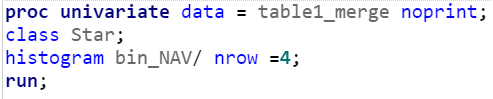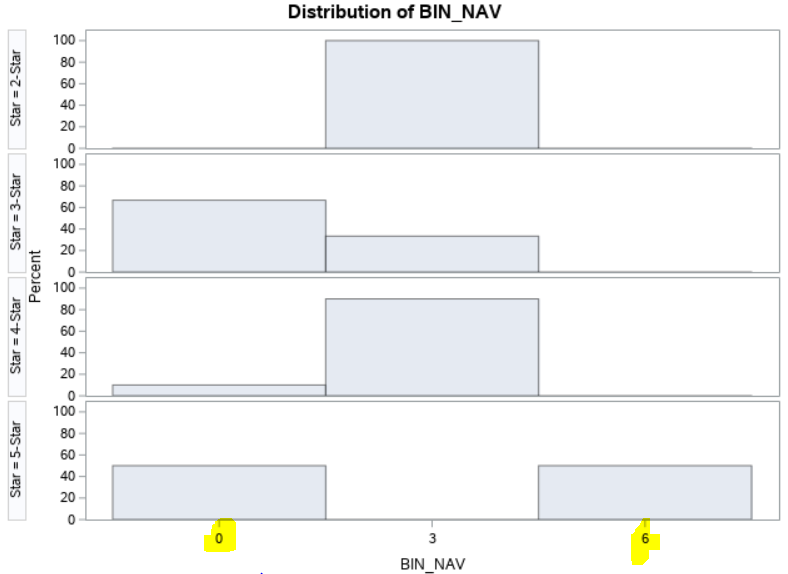## Histogram by class

Hello,

I have 2 Variables:  Star, bin_NAV.

- Star is the ordinal scale where its data included  2-star, 3-star, 4-star and 5-star.

- bin_NAV  is the ordinal scale where its data included  1, 2, 3, 4 and 5.

(bin_NAV is created from proc hpbin to bin the ratio scale variable, NAV, into 5 classes)

I want to build histogram classify by Star by the following procedure.The histogram show the value of bin_nav at 0 and 6, which are not in the data.  (See figure below)

What wrong is the code?

Thank You.1 ACCEPTED SOLUTION

Accepted Solutions

## Re: Histogram by class

UNIVARIATE doesn't know that you think the values of BIN_NAV are categories. It sees a numeric variable, considers it to be continuous, and bins them according to its default rule. You want to use the MIDPOINTS= option of the HISTOGRAM statement.

--
Paige Miller
2 REPLIES 2

## Re: Histogram by class

UNIVARIATE doesn't know that you think the values of BIN_NAV are categories. It sees a numeric variable, considers it to be continuous, and bins them according to its default rule. You want to use the MIDPOINTS= option of the HISTOGRAM statement.

--
Paige Miller